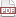### Synthesis via raking

25 November 12. [link]PDF version

I'd promised you some raking for missing data, but I'm putting that off for one more episode.

Last time ILast time showed you a table--only the margins mattered, so I'll just print those:

 - - 5 - - 15 10 10

Apophenia's raking setup doesn't take in pure margins like this; instead it wants a data set giving the value for eack cell in the table, that it will then sum into margins. So what should we fill in for the dashes? We just want something that matches the margins, and the exact values don't matter. If (1, 1, 1, 1) somehow fit, we'd run with it.

Of course, that question is exactly the raking question all over again: what is the closest table to the all-ones table that matches the given margins?

For today's sample code, let's make it happen. But before presenting the code, let me point out a trick I used to express the margins. Let us say that we have these three cells in the table:
(1, 1) = 0 ,
(1, 2) = 0 ,
(1, NaN) = 10 .
Then the sum for row 1 is ten. Also, if a cell is zero in the initial setup, and raking only re-scales cells, then that cell will remain zero throughout the procedure--zero times anything is zero. So if we start with an initial data set with no NaNs, then the (1, NaN) cell in the margin table is a sort of phantom cell that never gets used, and only affects the margin totals. Thus, the initial data listing sets the margins as we wish via the observations with NaNs in them, and the data cells themselves [like (1, 1) = 0 ] are just filler so the procedure doesn't get confused.

I need the NaN trick because my premise is that we don't have an already-extant data set that would produce the margin table. IRL, we typically have such a data set, so synthesis consists of finding the closest data set to the all-ones set that fits the original margins as written. At the end of the procedure, you have data that encapsulates the info from the margins of the margin table, but didn't use the individual cell values at all--synthetic data.

Here's the sample code for the NaN-using scenario, which should be reasonably legible. Again, if you have the requisites installed, you can cut/paste it to the command line and watch it go.

apop_text_to_db -O  -d="|" '-' margins na.db <<"----------"
row | col | weight
1 |  1 |   0
1 |  2 |   0
2 |  1 |   0
2 |  2 |   0
1 |  nan |   5
2 |  nan |   15
nan |  1 |   10
nan |  2 |   10
----------

cat <<"----------" > rake.c
#include <apop.h>

int main(){
apop_db_open("na.db");
apop_query("create table init as select row, col, 1 as val "
"from margins where row is not null and col is not null");
apop_data_show(
apop_rake(.margin_table="margins", .count_col="weight",
.contrasts=(char*[]){"row", "col"}, .contrast_ct=2,
.init_table="init", .init_count_col="val",)
);
}
----------

export CFLAGS="-g -Wall -O3  pkg-config --cflags apophenia"
export CC=c99 LDLIBS="pkg-config --libs apophenia"
make rake
./rake


###### Many dimensions

So far, I've been showing you only two-dimensional data, because that's what's easy to display on a static screen. Everything has obvious extension to margins in three, four, or twenty dimensions.

There are two intersting consequences to multiple dimensions, though. The first, which you shouldn't have to care about, is that high-dimensional matrices tend to be very sparse, so processing requires some care. Last time, I mentioned a table with 1.9 billion cells, of which 3 million were nonempty; you want a procedure that can work with only the 3 million cells.

The other is that we might want to fix to the margins not only single dimensions like rows and then columns, but higher-dimensional combinations of elements, such as specifying that all X, Z combinations must match a pre-set total. That is, we might want to hold fixed higher-dimensional slices.

If you are holding fixed a higher-dimensional slices, like X| Z as above, then the lower dimensional slices are also taken care of. If we fix the value of the sums for (X = 1, Z = 1) , (X = 1, Z = 2) , ..., (X = 1, Z = n) , then the total for X = 1 has to be fixed at the sum of these more detailed targets.

The people writing regression-type models like this because it makes sense that if you are regressing on the interaction' of X cross Z , then you would also want to include separate X and Z terms in there somewhere. Having mentioned that, I'm not going to go into too much detail on the use of raking as simulation of linear methods. I will keep the vocabulary, which uses the term contrast to refer to the X| Z combination.

Using Apophenia's syntax for this stuff (which is pretty similar to others, but for the C's compound literal (char*[]) cruft), we might have a call to the raking function like:

apop_rake(.margin_table="controltab",
.constrasts=(char*[]){"tract|age|sex", "race|ethnicity|tract"},
.contrast_ct=2);
`

This is going to generate synthetic data with the right tract| age| sex and tract| race| ethnicity distributions, beginning at the starting point of all ones (the default initial table when none is specified). Because only these two margins were set, we're evidently not worried about the age| race distribution or many other such combinations, which are likely to distort as the other contrasts are set.

At the extreme, if we have a 3-D table, and hold fixed the contrast X| Y| Z , then we are insisting that every cell in the table be fixed to the value given by the margin table. That is, we have zero degrees of freedom. If the margins are somehow inconsistent, then don't expect the raking procedure to converge.

Next time: structural zeros, and the promised missing data imputation.